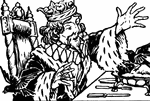King

Sing a Song of Sixpence; A pocket full of rye; Four-and-twenty blackbirds; Bak'd in a pie; When the…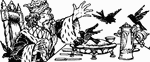King

Sing a Song of Sixpence; A pocket full of rye; Four-and-twenty blackbirds; Bak'd in a pie; When the…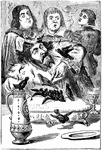King and Blackbirds

Sing a Song of Sixpence. And when the pie was opened, the birds began to sing.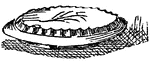Pie

Paste baked with something in it or under it, as apple, minced meat, etc.Fraction Pie Divided into Eighths

A circle divided into eighths.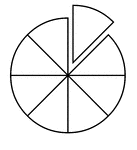Fraction Pie Divided into Eighths

A circle divided into eighths with one eighth separated.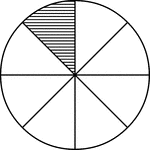Fraction Pie Divided into Eighths

A circle divided into eighths with one eighth shaded.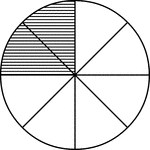Fraction Pie Divided into Eighths

A circle divided into eighths with two eighths shaded.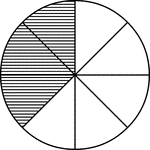Fraction Pie Divided into Eighths

A circle divided into eighths with three eighths shaded.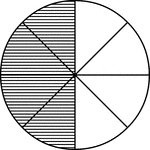Fraction Pie Divided into Eighths

A circle divided into eighths with four eighths shaded.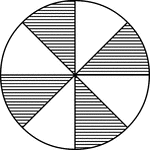Fraction Pie Divided into Eighths

A circle divided into eighths with four eighths shaded.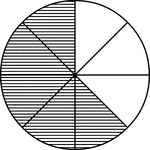Fraction Pie Divided into Eighths

A circle divided into eighths with five eighths shaded.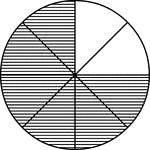Fraction Pie Divided into Eighths

A circle divided into eighths with six eighths shaded.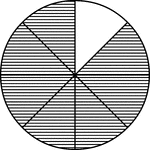Fraction Pie Divided into Eighths

A circle divided into eighths with seven eighths shaded.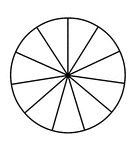Fraction Pie Divided into Elevenths

A circle divided into elevenths.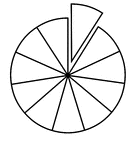Fraction Pie Divided into Elevenths

A circle divided into elevenths with one eleventh separated.Fraction Pie Divided into Elevenths

A circle divided into elevenths with one eleventh shaded.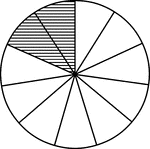Fraction Pie Divided into Elevenths

A circle divided into elevenths with two elevenths shaded.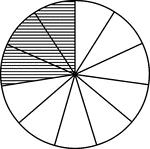Fraction Pie Divided into Elevenths

A circle divided into elevenths with three elevenths shaded.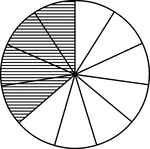Fraction Pie Divided into Elevenths

A circle divided into elevenths with four elevenths shaded.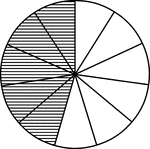Fraction Pie Divided into Elevenths

A circle divided into elevenths with five elevenths shaded.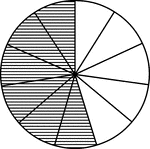Fraction Pie Divided into Elevenths

A circle divided into elevenths with six elevenths shaded.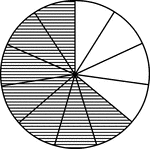Fraction Pie Divided into Elevenths

A circle divided into elevenths with seven elevenths shaded.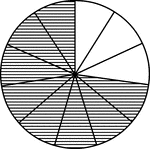Fraction Pie Divided into Elevenths

A circle divided into elevenths with eight elevenths shaded.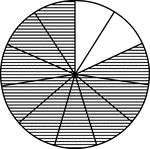Fraction Pie Divided into Elevenths

A circle divided into elevenths with nine elevenths shaded.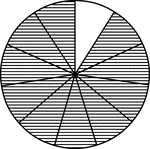Fraction Pie Divided into Elevenths

A circle divided into elevenths with ten elevenths shaded.Fraction Pie Divided into Fifths

A circle divided into fifths.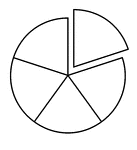Fraction Pie Divided into Fifths

A circle divided into fifths with one fifth separated.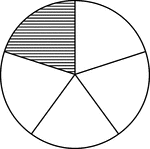Fraction Pie Divided into Fifths

A circle divided into fifths with one fifth shaded.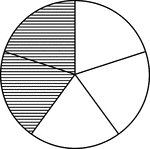Fraction Pie Divided into Fifths

A circle divided into fifths with two fifths shaded.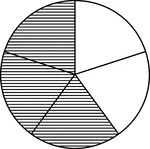Fraction Pie Divided into Fifths

A circle divided into fifths with three fifths shaded.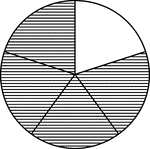Fraction Pie Divided into Fifths

A circle divided into fifths with four fifths shaded.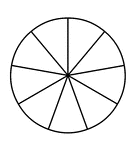Fraction Pie Divided into Ninths

A circle divided into ninths.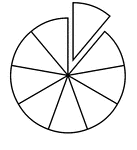Fraction Pie Divided into Ninths

A circle divided into ninths with one ninth separated.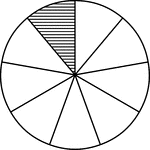Fraction Pie Divided into Ninths

A circle divided into ninths with one ninth shaded.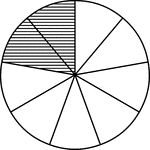Fraction Pie Divided into Ninths

A circle divided into ninths with two ninths shaded.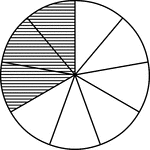Fraction Pie Divided into Ninths

A circle divided into ninths with three ninths shaded.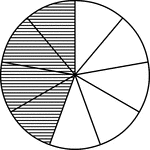Fraction Pie Divided into Ninths

A circle divided into ninths with four ninths shaded.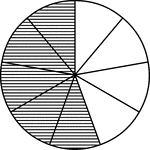Fraction Pie Divided into Ninths

A circle divided into ninths with five ninths shaded.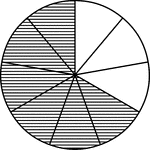Fraction Pie Divided into Ninths

A circle divided into ninths with six ninths shaded.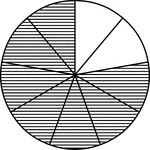Fraction Pie Divided into Ninths

A circle divided into ninths with seven ninths shaded.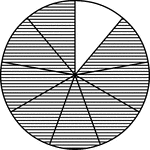Fraction Pie Divided into Ninths

A circle divided into ninths with eight ninths shaded.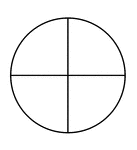Fraction Pie Divided into Quarters

A circle divided into quarters.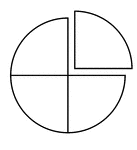Fraction Pie Divided into Quarters

A circle divided into quarters with one quarter separated.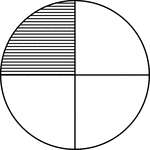Fraction Pie Divided into Quarters

A circle divided into quarters with one quarter shaded.Fraction Pie Divided into Quarters

A circle divided into quarters with two quarters shaded.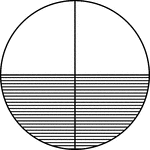Fraction Pie Divided into Quarters

A circle divided into quarters with two quarters shaded.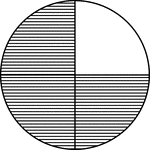Fraction Pie Divided into Quarters

A circle divided into quarters with three quarters shaded.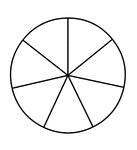Fraction Pie Divided into Sevenths

A circle divided into sevenths.